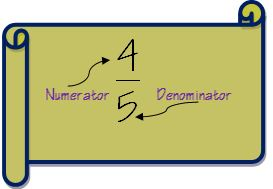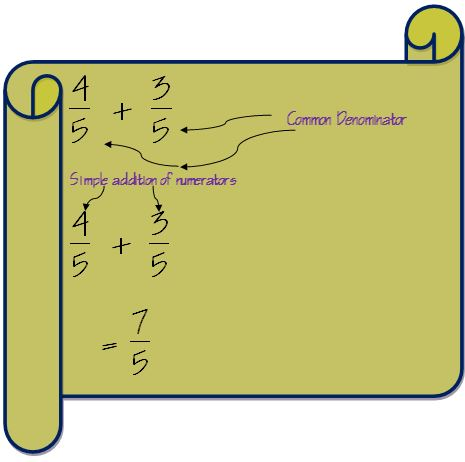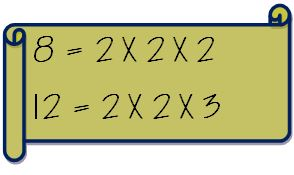# Suggest a simpler way to add fractions

Well fractions can be really scary, I must admit. To add fractions is scarier even. It seems like they are the starting point of the scariness of the world of mathematics. Until you realize that dealing with fractions is also as simple as dealing with natural numbers, if you know the trick.

### Method 1

1. First let us understand the terminology of fractions before moving to steps to add fractions. A fraction has two parts- numerator and denominator. If you really want to become a scholar in Fractions you may visit the Fraction page on Wikipedia.

Numerator is the number above the line in a fraction and denominator is the number below the line.The first step to add fractions is to check the denominators of the two fractions. It the denominator for both the fractions is same then addition becomes very simple.

In such a case we just have to add the numerators and denominator will remain the same.But if the denominators are not same then we need to make the denominators of both the fractions same. The method to do is to find the least common multiple of the denominators.

#### How to find least common multiple

Say we have to find LCM of two numbers 8 and 12.

First write down the prime factors of the two numbers separately.Now take out the common factors and multiply it with the non-common factors. So the LCM of 8 and 12 will be calculated as follow.#### Add fractions with different denominators

Say we have two fractions 3/8 and 5/12

The steps are:

1. Check if the denominators are same for both the fractions. If the denominators are same then we follow the method mentioned above. In our case they are different.2. Find out the LCM of the denominator. As illustrated above the LCM for 8 and 12 is 24.

3. Make both the denominators same as the LCM by multiplying with suitable numbers.4. If we have multiplied the denominator by a number then we also need to multiply the numerator by the same number as well to keep the fraction intact.5. By performing these steps we get two fractions whose denominators are same. So we just have to add the numerators like we did in our earlier example.6. We get the result as 19/24

7. We have to check if there is any common factor between the numerator and denominator or not after addition is performed.

8. If there is no common factor then we can report the result as our final answer.### Method 2

This method will also give us the desired result but it is not suggested for fifth grade students who want to learn to add fractions.

1. Convert both the fraction into numbers by dividing numerator by denominator2. Now it is simple addition of two numbers